# 0. 简介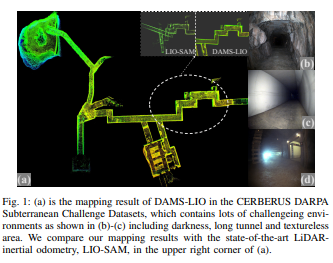# 1. 文章贡献

1. 提出了一种基于退化感知和模块化传感器融合的轻量级激光雷达-惯性测程系统(DAMS-LIO)，该系统能够在极端环境下进行鲁棒和准确的状态估计，在计算资源有限的情况下，为机器人执行复杂探测任务提供了明显的优势。

2. 提出了一种新的传感器融合方法，将激光雷达和其他里程计的信息完全融合，仅在检测到退化时，将激光雷达点和其他里程计的相对位姿作为更新过程中的测量值。

3. 基于CRLB定理进行了理论分析，以量化性能，并证明了所提出的传感器融合方法的高精度。

4. 在模拟和真实数据集上的大量实验验证了我们方法的鲁棒性和准确性。

# 2. 系统架构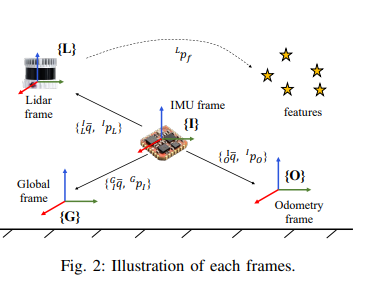^Lp表示每个点相对于激光雷达坐标系的位置。一些重要且常用符号的定义在表格I中给出。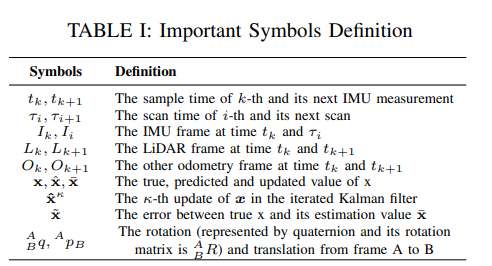### A. 状态向量（这部分内容比较通用）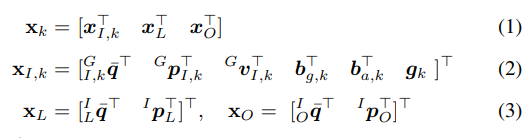^Gv_I是IMU在全局坐标系下的速度，b_{g,k}b_{a,k}分别表示陀螺仪和加速度计的偏差，g是在{G}坐标系下的重力向量，x_L = {^I_L \bar{q}, ^Ip_L}x_O = {^I_O \bar{q}, ^Ip_O}表示从其他里程计坐标系{O}和LiDAR坐标系{L}到IMU坐标系{I}的变换。

### B. IMU传播（同理IMU的传播）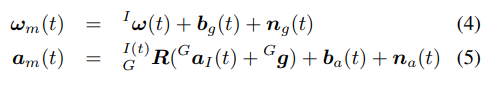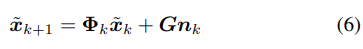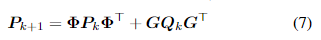### C. 测量模型（比较重点的知识）

1）LiDAR测量：对于来自LiDAR的测量，我们将其建模为中的模型。在对在τi时间采样的扫描进行运动补偿后，我们将局部LiDAR坐标系中的第j个点定义为^Lp_j。通过向后传播，该点可以更改为与t_k处IMU测量对应的扫描结束测量。同时，由于每个点应该位于地图中的小平面区域上，我们可以得到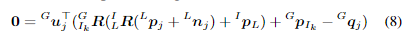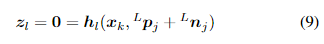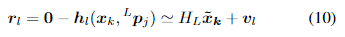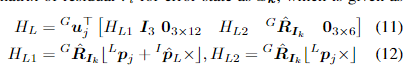2) 另一种里程表测量：由于每个里程表的发布频率不同，我们执行线性插值以获取估计状态在时间 t_kt_{k+1} 时的其他里程表姿态，并将它们的坐标系表示为 O_{k−1}O_k。我们将从 A 到 B 的变换矩阵 ^AT_B 定义为 ^AT_B = [^AR_B ,^Ap_B ; 0_{3×3}, 1]。然后根据图 2，我们有以下变换关系：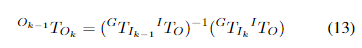v_r∈N(0,R_r)v_p∈N(0,R_p)$$是相应的高斯噪声。因此，根据公式（14）和（15），我们可以得到旋转和平移残差的误差状态的雅可比矩阵。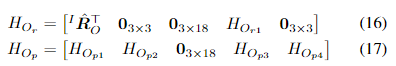其中：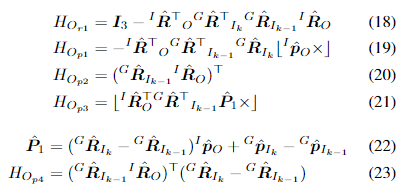### D. 变形感知更新（比较重点的知识） 与类似，我们有以下误差状态：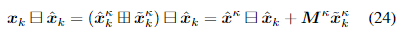\boxplus/\boxminus表示Lie群中的加减运算符。M_\kappa(\hat{x}^\kappa_k \boxplus \tilde{x}^\kappa_k)\boxminus \hat{x}_k相对于\tilde{x}^\kappa_k的偏导数，以零为值进行评估。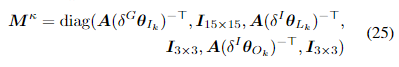其中，A(\cdot)定义在中，\delta^X\theta_Y = ^X\hat{R}^\kappa _Y \boxminus ^X\hat{R}_Y。根据(24)，并将其带入上述的一阶逼近测量模型中，问题可以总结为：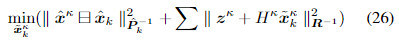为了避免位姿测量噪声影响LiDAR里程计的良好性能并在退化条件下增强里程计鲁棒性，我们采用了灵活的更新方案。理论上，对于退化维度对应的特征值，它们是精确为零的，但由于数据噪声和有限的计算精度，实际计算中通常是一个小值。因此，我们首先计算Hessian矩阵H^T_L H_L的特征值{λ_i}，并参考中的启发式方法确定场景的几何特征。如果特征值小于阈值，则可以推断存在退化。**如果LIO处于良好状态并且假设我们有m个LiDAR测量，则z^κ = z^κ_l = [z^κ_{l1}, . . . , z^κ_{lm}]^T, H = H^κ_L = [H^κ_{l1}, . . . , H^κ_{lm}]^TR = R_L = diag(R_{l1}, . . . , R_{lm})。否则，如果检测到退化，z^κ = [z^κ_l，z^κ_r，z^κ_p ]H = [H^κ_L，H^κ_{O_r}，H^κ_{O_p}]^TR = diag(R_L，R_{O_r}，R_{O_p})**。然后，我们可以执行中相同的迭代卡尔曼滤波更新。 # 3. CramÉr-rao下界定理(重点知识) 在本节中，我们为读者提供有关所提出的融合方法的更深入见解，并使用CraméR-Rao下限（CRLB）将其与其他纯激光雷达方法的精度进行比较。 CRLB是一个估计器方差的下限，通常用于评估数据融合方法的性能 。根据，CRLB通过取费舍尔信息矩阵的逆来计算：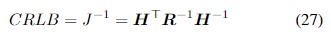其中，HR分别对应于第III节中的测量雅可比矩阵和协方差矩阵。CRLB越小，系统在理论上就可以达到更高的精度。为了分析需要，我们需要以下引理。 引理1：正定矩阵的逆矩阵也是正定的。 引理2：如果存在一个实数非奇异矩阵B使得A = BB^T，则实对称矩阵A是正定的。 引理3：设A为正定矩阵，Bm×n的实数矩阵。如果B的秩：r(B) = n，则B^TAB是正定的。 基于上述理论，我们分别计算并比较不同方法的CRLB。 1. 纯基于LiDAR的方法：由于纯基于LiDAR的方法不使用里程计位姿测量，因此将与里程计相关的外参从状态向量中移除。此外，为了简化计算，我们忽略对应于$$H$$中为零向量的变量。因此，基于（11）和（12），纯基于LiDAR的测量雅可比矩阵可以重写为：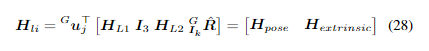我们将式(28)代入式(27)，并表示为一个分块矩阵的形式，具体如下：由于 LiDAR 噪声的协方差矩阵是实对称矩阵，且$$H_{li}$$的秩等于它的行数，我们可以推断出$$J_{li}^{-1}$$是正定矩阵，表示为$$J_{li}^{-1}>0$$。因此，根据分块矩阵的逆公式，我们可以轻松地得到对应于估计位姿的部分，表示为：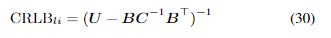2. 姿态融合方法：按照上述过程，我们得到的测量雅可比矩阵如下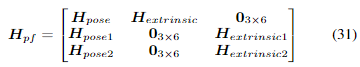然后我们将公式(31)代入(27)并将其表示为与(29)相同的块矩阵形式：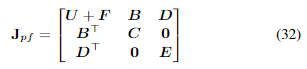然后，我们边缘化里程计外参相关的变量，得到变量的边缘化费舍尔信息矩阵$$J_{pf_{mar}}$$。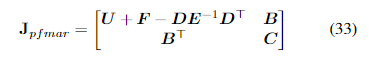接着，根据公式（30），估计位姿的对应 CRLB 为：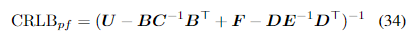3. 比较：考虑仅使用里程计姿态测量的情况（即状态向量中没有 LiDAR 和 IMU 之间的外参变量），则 Fisher 信息矩阵为：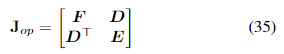根据这种情况，$$(F − DE^{−1}D^T)^{−1}$$是对估计姿态对应的信息矩阵，因此显然是正定的。根据引理1，我们可以得出$$(F − DE^{−1}D^T) > 0$$。根据，我们有：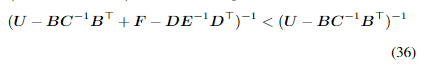然后，可以验证$$CRLB_{pf} < CRLB_{li}。也就是说，将姿态测量和LiDAR点融合的方法，在姿态估计的协方差下有一个更小的下限，比仅使用LiDAR点的方法更优。将上述分析代入应用场景，表明我们的传感器融合方法充分利用了比那些将里程计信息用作初始值的方法更多的里程计测量信息，因此在姿态估计方面实现了更好的准确性。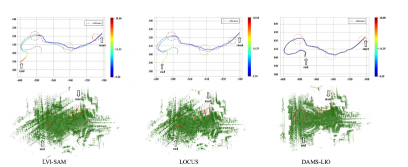# 4. 参考链接

https://mp.weixin.qq.com/s/T9xE0LJ3iYX8oEGwFtv9hQ